Overview: Periodic Classification of Elements - 2

# Overview: Periodic Classification of Elements - 2 - Science Class 10

## Position of Elements in the Modern Periodic Table

Modem Periodic Table has 18 vertical columns named as ‘groups’ and 7 horizontal rows known as ‘periods’. Elements with same number of valence electrons are placed in the same group. In other words, a group in a periodic table signifies an identical outer shell electronic configuration.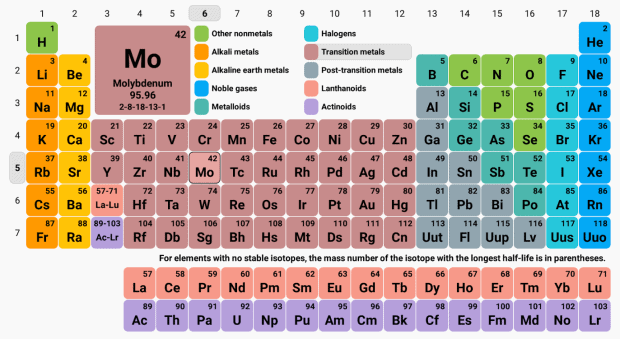Activity: Explain the similarity of group 1 elements.

Consider the elements lithium, sodium and potassium of group 1.

Their electronic configuration may be written as 6: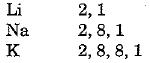Thus, the outermost or valence shell in all three cases contains 1 electron. These elements have been placed in the same group because of this similarity.

Consider the elements Li, Be, B, C, N, O, F and Ne which are present in the second period. The distribution of electrons in these elements can be written as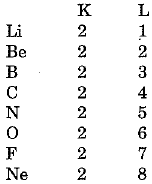We find that the number of electrons in the outermost (valence) shell in the second period elements is not the same. But all these elements contain the same number of shells Le., two. Also we observe that the valence electrons increase successively by one unit. In other words, elements with same number of occupied shells are placed in the same period. Na, Mg, Al, Si, P, S, Cl and Ar belong to the third period because the electrons in these atoms are filled in the three shells, K, L and M.
Maximum number of electrons that can be filled in a shell is given by the 2n2 where n is shell number.
For K-shell n = 1 or 2n2 = 2
Hence, first period has 2 elements.
For L-shell n = 2 or 2n2 = 8
Hence, second period has 8 elements.
For M-shell n = 3 or 2n2, - 1
But the outermost shell can have 8 electrons.
Therefore, the third period can have 8 elements.
The position of an element in the periodic table tells us about its chemical reactivity. Valence electrons determine the kind and number of bonds formed by the element. That is why, Mendeleev made it a basis for classifying elements.

### Valency

Valency is given by the number of electrons present in the valence shell i.e., outermost shell.
Activity: To determine the valency of an element.
Let us calculate the valency of Mg with atomic number 12 and valency of sulphur with atomic number 16.
Mg  2, 8, 2
Thus, Mg has a valency of 2.
S  2, 8, 6
Sulphur can form upto 6 bonds.
We observe that valency increases on going from left to right in a period and remains constant in moving from top to bottom in a group.

### Atomic size

Atomic size (or atomic radius) may be visualised as the distance between the centre of the atom and the outermost shell of the isolated atom. Atomic radius of hydrogen is 37 pm (1 pm - 10-12 m).
Activity: Study the variation in atomic radii of elements in a period.
The atomic radii of the elements of second period are given below :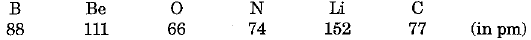(i) They can be arranged in decreasing order of atomic radius as under :
Li > Be > B > C > N > O
(ii) We observe that the above order is the order of arrangement of elements in the second period in the periodic table.
(iii) Lithium has the largest and oxygen has the smallest atom.
Atomic radius decreases as we go from left to right in a period. We can ascribe it to increase in nuclear charge which tends to pull the electron closer to the nucleus so that the size of the atom is reduced.
Activity: Study the variation in atomic size of elements in a group.
The atomic radii of the elements of first group are given below :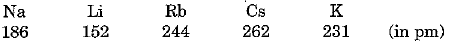(i) The elements can be arranged in increasing order of their atomic radii as under :
Li < Na < K < Rb < Cs
(ii) Lithium has the smallest atoms and caesium has the largest atoms.
(iii) Atomic size increases as we move down the group. This is because new shells are being added as we move down and this increases the distance between the nucleus and outermost electrons. There is an increase in the radius in spite of increase in nuclear charge.

### Properties

Activity: Classify the elements of third period into metals and non-metals.
The elements of the third period are:
Na Mg Al Si P S Cl Ar
(i) We find that Na, Mg and A1 have tendency to lose electrons, therefore, they behave as metal. Elements like P, S and Cl have a tendency to gain electrons. Therefore, they behave as non-metals.
(ii) Metals occupy the left hand side of the periodic table
(iii) Non-metals occupy right hand side of the periodic table.
In the middle we have silicon which is classified as a semi-metal or metalloid because it exhibits some properties of both metals and non-metals.
Activity: Study the tendency of elements to lose electrons in a group.
(i) The effective nuclear charge acting on valence electrons decreases while moving down the group because the outermost electrons are farther away from the nucleus. Therefore, tendency to lose electrons increases down the group.
(ii) The effective nuclear charge acting on the valence shell electrons increases across a period, because of increased nuclear charge and decreasing size of the atom. Therefore, the tendency to lose electrons decreases in going from left to right in a period. Hence, metallic character decreases across the period but increases down the group.

The document Overview: Periodic Classification of Elements - 2 | Science Class 10 is a part of the Class 10 Course Science Class 10.
All you need of Class 10 at this link: Class 10

## Science Class 10

78 videos|509 docs|153 tests

## FAQs on Overview: Periodic Classification of Elements - 2 - Science Class 10

 1. What is the position of elements in the modern periodic table?Ans. The elements in the modern periodic table are arranged in order of their increasing atomic number. They are organized into rows called periods and columns called groups based on their similar chemical properties.
 2. What are some trends in the periodic table?Ans. There are several trends in the periodic table. One such trend is the periodic recurrence of similar properties as you move across a period from left to right. Another trend is the increase in atomic size as you move down a group. Additionally, the elements on the left side of the periodic table tend to be metals, while those on the right side tend to be non-metals.
 3. How is the periodic table classified?Ans. The periodic table is classified into periods and groups. Periods are the horizontal rows, and there are a total of seven periods. Groups are the vertical columns, and there are 18 groups in the periodic table. Elements in the same group share similar chemical properties.
 4. What is the significance of the atomic number in the periodic table?Ans. The atomic number is the number of protons in the nucleus of an atom. In the periodic table, elements are arranged in order of increasing atomic number. The atomic number determines the element's identity and plays a crucial role in determining the element's properties and its position in the periodic table.
 5. Why are elements with similar properties placed in the same group in the periodic table?Ans. Elements with similar properties are placed in the same group in the periodic table because they have the same number of valence electrons. Valence electrons are responsible for an element's chemical behavior and determine its reactivity and bonding patterns. Therefore, elements in the same group tend to exhibit similar chemical properties.

## Science Class 10

78 videos|509 docs|153 testsExplore Courses for Class 10 examSignup to see your scores go up within 7 days! Learn & Practice with 1000+ FREE Notes, Videos & Tests.
10M+ students study on EduRev
Track your progress, build streaks, highlight & save important lessons and more!
Related Searches

,

,

,

,

,

,

,

,

,

,

,

,

,

,

,

,

,

,

,

,

,

;